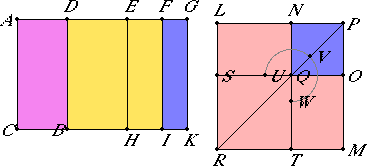# Proposition 93

If an area is contained by a rational straight line and a third apotome, then the side of the area is a second apotome of a medial straight line.

Let the area AB be contained by the rational straight line AC and the third apotome AD.

I say that the side of the area AB is a second apotome of a medial straight line.X.Def.III.3

Let DG be the annex to AD. Then AG and GD are rational straight lines commensurable in square only, and neither of the straight lines AG and GD is commensurable in length with the rational straight line AC set out, while the square on the whole AG is greater than the square on the annex DG by the square on a straight line commensurable with AG.

X.17

Since, then, the square on AG is greater than the square on GD by the square on a straight line commensurable with AG, therefore, if there is applied to AG a parallelogram equal to the fourth part of the square on DG and deficient by a square figure, then it divides it into commensurable parts.

Bisect DG at E, apply to AG a parallelogram equal to the square on EG and deficient by a square figure, and let it be the rectangle AF by FG. Draw EH, FI, and GK through the points E, F, and G parallel to AC.

Then AF and FG are commensurable. Therefore AI is also commensurable with FK.

X.15

Since AF and FG are commensurable in length, therefore AG is also commensurable in length with each of the straight lines AF and FG.

X.13

But AG is rational and incommensurable in length with AC, so that AF and FG are so also.

X.21

Therefore each of the rectangles AI and FK is medial.

X.15

Again, since DE is commensurable in length with EG, therefore DG is also commensurable in length with each of the straight lines DE and EG.

But GD is rational and incommensurable in length with AC, therefore each of the straight lines DE and EG is also rational and incommensurable in length with AC. Therefore each of the rectangles DH and EK is medial.

Since AG and GD are commensurable in square only, therefore AG is incommensurable in length with GD.

X.13

But AG is commensurable in length with AF, and DG with EG, therefore AF is incommensurable in length with EG.

But AF is to EG as AI is to EK, therefore AI is incommensurable with EK.

VI.26

Now construct the square LM equal to AI, and subtract NO, equal to FK, about the same angle with LM. Then LM and NO are about the same diameter.

Let PR be their diameter, and draw the figure.

VI.17

Now, since the rectangle AF by FG equals the square on EG, therefore AF is to EG as EG is to FG.

But AF is to EG as AI is to EK, and EG is to FG as EK is to FK, therefore AI is to EK as EK is to FK. Therefore EK is a mean proportional between AI and FK.

But MN is also a mean proportional between the squares LM and NO, and AI equals LM, and FK equals NO, therefore EK also equals MN.

But MN equals LO, and EK equals DH, therefore the whole DK also equals the gnomon UVW and NO.

But AK equals the sum of LM and NO, therefore the remainder AB equals ST, that is, to the square on LN. Therefore LN is the side of the area AB.

I say that LN is a second apotome of a medial straight line.

Since AI and FK were proved medial, and equal the squares on LP, therefore each of the squares on LP and PN is also medial. Therefore each of the straight lines LP and PN is medial.

Since AI is commensurable with FK, therefore the square on LP is also commensurable with the square on PN.

Again, since AI was proved incommensurable with EK, therefore LM is also incommensurable with MN, that is, the square on LP with the rectangle LP by PN, so that LP is also incommensurable in length with PN.

Therefore LP and PN are medial straight lines commensurable in square only.

I say next that they also contain a medial rectangle.

Since EK was proved medial, and equals the rectangle LP by PN, therefore the rectangle LP by PN is also medial, so that LP and PN are medial straight lines commensurable in square only which contain a medial rectangle.

X.75

Therefore LN is a second apotome of a medial straight line, and it is the side of the area AB.

Therefore the side of the area AB is a second apotome of a medial straight line.

Therefore, if an area is contained by a rational straight line and a third apotome, then the side of the area is a second apotome of a medial straight line.

Q.E.D.

## Guide

This proposition is used in X.110.